Select PagePrintable version

The missing numbers are between 1 & 9.  The three numbers in each row, when multiplied or times together, equals the totals on the right, and the columns, when multiplied together, equals the totals along the bottom.  The products of the two diagonals are also given.  Solve the puzzle by finding all of the missing numbers that satisfy all of the totals as shown.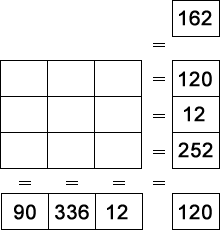### Solution### Solution explanation

Letters have been inserted in the original puzzle example, so that the unknown values can be determined.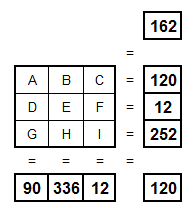We can write the following 8 equations for this puzzle.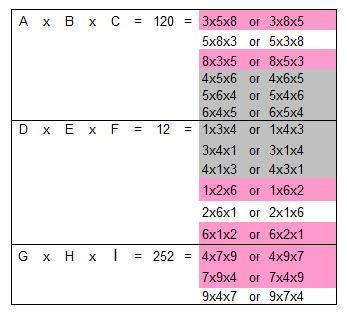Note: G, H & I can only = the values 4, 7 or 9. Therefore; A, B, C, D, E or F can not = 4, 7 or 9 and after futher evaluation of the equations above; A, B & C can only be = to 3, 5 or 8 and D, E & F can only be = to 1, 2 or 6.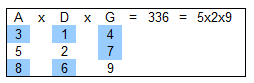Comparing all possible combinations of A, D & G, it must be that A = 5, D = 2 & G = 9.

After further evaluation of the equations above:Therefore, comparing all possible combinations of B, E & H, it must be that B = 8, E = 6 & H = 7 and C = 3, F = 1 & I = 4.

Checking the final answers in the remaining 3 equations: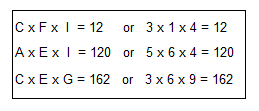The puzzle is solved!## Feedback

There are more than one way of doing these puzzles and may well be more than one answer.  Please let me and others know what alternatives you find by commenting below.  We also welcome general comments on the subject and any feedback you’d like to give.   If you have a question that need a response from me or you would like to contact me privately, please use the contact form.

## Get more puzzles!

If you’ve enjoyed doing the puzzles, consider ordering the book; 150+ of the best puzzles in a handy pocket sized format. Click here for full details.